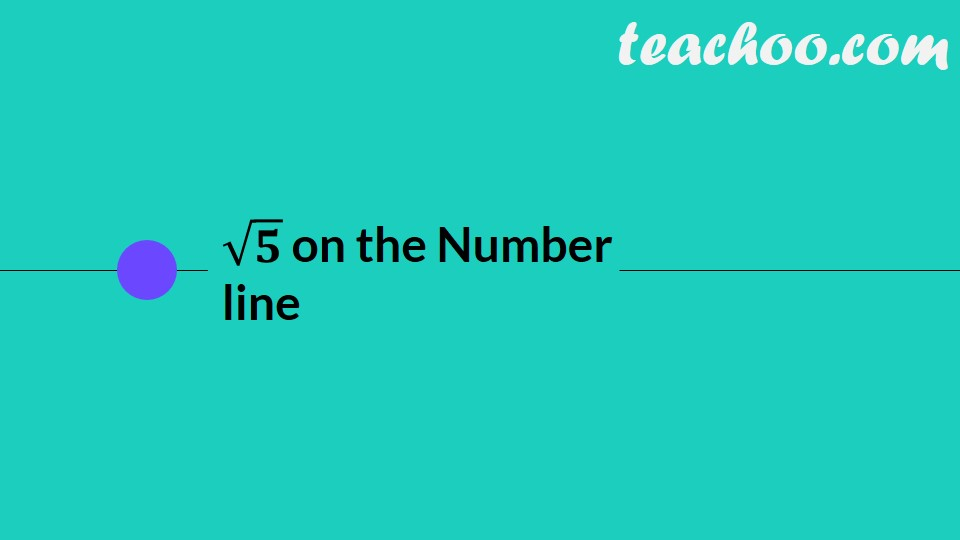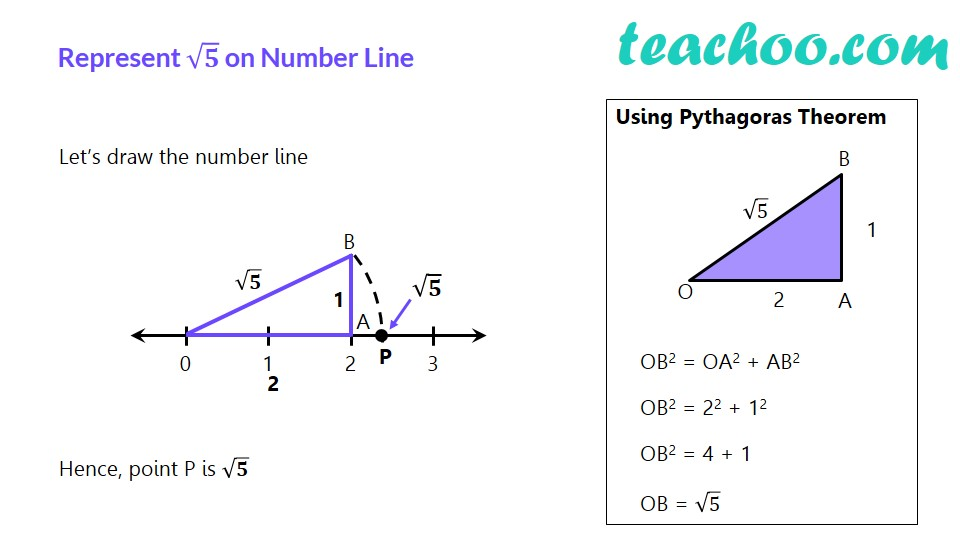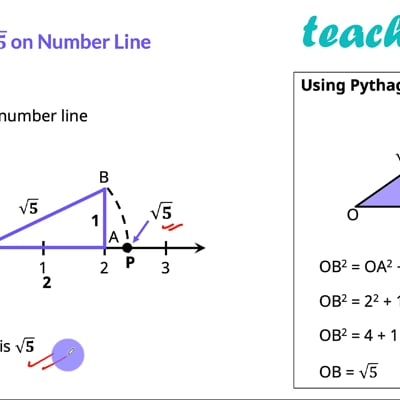Chapter 1 Class 9 Number Systems

Class 9
Important Questions for Exam - Class 9This video is only available for Teachoo black users

### Transcript

√𝟓 on the Number line Represent √𝟓 on Number Line Let’s draw the number line Hence, point P is √𝟓 Using Pythagoras Theorem OB2 = OA2 + AB2 OB2 = 22 + 12 OB2 = 4 + 1 OB = √5 Ex1.2,3 Locate √5 on the number line For drawing √5 we consider Pythagoras theorem. Using Pythagoras theorem Hypotenuse2 = Base2 + Height2 Hypotenuse = √("Base2 + Height2" ) Hypotenuse = √(2^2+1^2 ) Hypotenuse = √(4+1) Hypotenuse = √5 Point P is the point of √5